xDivergenceEncyclopedia
In vector calculus, divergence is a vector operator that measures the magnitude of a vector field
Vector field
In vector calculus, a vector field is an assignmentof a vector to each point in a subset of Euclidean space. A vector field in the plane for instance can be visualized as an arrow, with a given magnitude and direction, attached to each point in the plane...

's source or sink at a given point, in terms of a signed scalar. More technically, the divergence represents the volume density of the outward flux
Flux
In the various subfields of physics, there exist two common usages of the term flux, both with rigorous mathematical frameworks.* In the study of transport phenomena , flux is defined as flow per unit area, where flow is the movement of some quantity per time...

of a vector field from an infinitesimal volume around a given point. For example, consider air as it is heated or cooled. The relevant vector field for this example is the velocity
Velocity
In physics, velocity is speed in a given direction. Speed describes only how fast an object is moving, whereas velocity gives both the speed and direction of the object's motion. To have a constant velocity, an object must have a constant speed and motion in a constant direction. Constant ...

of the moving air at a point. If air is heated in a region it will expand in all directions such that the velocity field points outward from that region. Therefore the divergence of the velocity field in that region would have a positive value, as the region is a source. If the air cools and contracts, the divergence is negative and the region is called a sink.

## Definition of divergence

In physical terms, the divergence of a three dimensional vector field is the extent to which the vector field flow behaves like a source or a sink at a given point. It is a local measure of its "outgoingness"—the extent to which there is more exiting an infinitesimal region of space than entering it. If the divergence is nonzero at some point then there must be a source or sink at that position. (Note that we are imagining the vector field to be like the velocity vector field of a fluid (in motion) when we use the terms flow, sink and so on.)

More rigorously, the divergence of a vector field F at a point p is defined as the limit of the net flow of F across the smooth boundary of a three dimensional region V divided by the volume of V as V shrinks to p. Formally,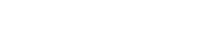where |V | is the volume of V, S(V) is the boundary of V, and the integral is a surface integral
Surface integral
In mathematics, a surface integral is a definite integral taken over a surface ; it can be thought of as the double integral analog of the line integral...

with n being the outward unit normal to that surface. The result, div F, is a function of the location p. From this definition it also becomes explicitly visible that div F can be seen as the source density of the flux of F.

In light of the physical interpretation, a vector field with constant zero divergence is called incompressible or solenoidal – in this case, no net flow can occur across any closed surface.

The intuition that the sum of all sources minus the sum of all sinks should give the net flow outwards of a region is made precise by the divergence theorem
Divergence theorem
In vector calculus, the divergence theorem, also known as Gauss' theorem , Ostrogradsky's theorem , or Gauss–Ostrogradsky theorem is a result that relates the flow of a vector field through a surface to the behavior of the vector field inside the surface.More precisely, the divergence theorem...

.

### Application in Cartesian coordinates

Let x, y, z be a system of Cartesian coordinates on a 3-dimensional Euclidean space
Euclidean space
In mathematics, Euclidean space is the Euclidean plane and three-dimensional space of Euclidean geometry, as well as the generalizations of these notions to higher dimensions...

, and let ijk be the corresponding basis
Basis (linear algebra)
In linear algebra, a basis is a set of linearly independent vectors that, in a linear combination, can represent every vector in a given vector space or free module, or, more simply put, which define a "coordinate system"...

of unit vectors.

The divergence of a continuously differentiable vector field
Vector field
In vector calculus, a vector field is an assignmentof a vector to each point in a subset of Euclidean space. A vector field in the plane for instance can be visualized as an arrow, with a given magnitude and direction, attached to each point in the plane...

F = U i + V j + W k is equal to the scalar
Scalar (mathematics)
In linear algebra, real numbers are called scalars and relate to vectors in a vector space through the operation of scalar multiplication, in which a vector can be multiplied by a number to produce another vector....

-valued function: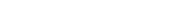Although expressed in terms of coordinates, the result is invariant under orthogonal transformation
Orthogonal matrix
In linear algebra, an orthogonal matrix , is a square matrix with real entries whose columns and rows are orthogonal unit vectors ....

s, as the physical interpretation suggests.

The common notation for the divergence ∇·F is a convenient mnemonic, where the dot denotes an operation reminiscent of the dot product
Dot product
In mathematics, the dot product or scalar product is an algebraic operation that takes two equal-length sequences of numbers and returns a single number obtained by multiplying corresponding entries and then summing those products...

: take the components of ∇ (see del
Del
In vector calculus, del is a vector differential operator, usually represented by the nabla symbol \nabla . When applied to a function defined on a one-dimensional domain, it denotes its standard derivative as defined in calculus...

), apply them to the components of F, and sum the results. Because applying an operator is different from multiplying the components, this is considered an abuse of notation
Abuse of notation
In mathematics, abuse of notation occurs when an author uses a mathematical notation in a way that is not formally correct but that seems likely to simplify the exposition or suggest the correct intuition . Abuse of notation should be contrasted with misuse of notation, which should be avoided...

.

The divergence of a continuously differentiable tensor
Tensor
Tensors are geometric objects that describe linear relations between vectors, scalars, and other tensors. Elementary examples include the dot product, the cross product, and linear maps. Vectors and scalars themselves are also tensors. A tensor can be represented as a multi-dimensional array of...

field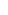is:### Cylindrical coordinates

For a vector expressed in cylindrical coordinates as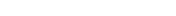where ea is the unit vector in direction a, the divergence is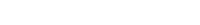### Spherical coordinates

In spherical coordinates, with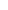the angle with the z axis and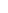the rotation around the z axis, the divergence reads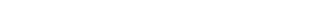## Decomposition theorem

It can be shown that any stationary flux v(r) which is at least two times continuously differentiable in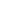and vanishes sufficiently fast for can be decomposed into an irrotational part E(r) and a source-free part B(r). Moreover, these parts are explicitly determined by the respective source-densities (see above) and circulation densities (see the article Curl):

For the irrotational part one has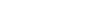with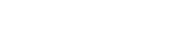The source-free part, B, can be similarly written: one only has to replace the scalar potential Φ(r) by a vector potential A(r) and the terms −Φ by +×A, and finally the source-density
by the circulation-density ×v.

This "decomposition theorem" is in fact a by-product of the stationary case of electrodynamics. It is a special case of the more general Helmholtz decomposition
Helmholtz decomposition
In physics and mathematics, in the area of vector calculus, Helmholtz's theorem, also known as the fundamental theorem of vector calculus, states that any sufficiently smooth, rapidly decaying vector field in three dimensions can be resolved into the sum of an irrotational vector field and a...

which works in dimensions greater than three as well.

## Properties

The following properties can all be derived from the ordinary differentiation rules of calculus
Calculus
Calculus is a branch of mathematics focused on limits, functions, derivatives, integrals, and infinite series. This subject constitutes a major part of modern mathematics education. It has two major branches, differential calculus and integral calculus, which are related by the fundamental theorem...

. Most importantly, the divergence is a linear operator, i.e.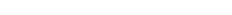for all vector fields F and G and all real number
Real number
In mathematics, a real number is a value that represents a quantity along a continuum, such as -5 , 4/3 , 8.6 , √2 and π...

s a and b.

There is a product rule
Product rule
In calculus, the product rule is a formula used to find the derivatives of products of two or more functions. It may be stated thus:'=f'\cdot g+f\cdot g' \,\! or in the Leibniz notation thus:...

of the following type: if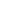is a scalar valued function and F is a vector field, then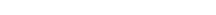or in more suggestive notation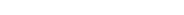Another product rule for the cross product
Cross product
In mathematics, the cross product, vector product, or Gibbs vector product is a binary operation on two vectors in three-dimensional space. It results in a vector which is perpendicular to both of the vectors being multiplied and normal to the plane containing them...

of two vector fields F and G in three dimensions involves the curl and reads as follows: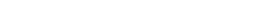or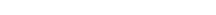The Laplacian of a scalar field
Scalar field
In mathematics and physics, a scalar field associates a scalar value to every point in a space. The scalar may either be a mathematical number, or a physical quantity. Scalar fields are required to be coordinate-independent, meaning that any two observers using the same units will agree on the...

is the divergence of the field's gradient: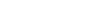The divergence of the curl of any vector field (in three dimensions) is equal to zero: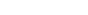If a vector field F with zero divergence is defined on a ball in R3, then there exists some vector field G on the ball with F = curl(G). For regions in R3 more complicated than this, the latter statement might be false (see Poincaré lemma). The degree of failure of the truth of the statement, measured by the homology
Homology (mathematics)
In mathematics , homology is a certain general procedure to associate a sequence of abelian groups or modules with a given mathematical object such as a topological space or a group...

of the chain complex
Chain complex
In mathematics, chain complex and cochain complex are constructs originally used in the field of algebraic topology. They are algebraic means of representing the relationships between the cycles and boundaries in various dimensions of some "space". Here the "space" could be a topological space or...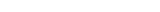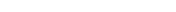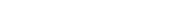(where the first map is the gradient, the second is the curl, the third is the divergence) serves as a nice quantification of the complicatedness of the underlying region U. These are the beginnings and main motivations of de Rham cohomology
De Rham cohomology
In mathematics, de Rham cohomology is a tool belonging both to algebraic topology and to differential topology, capable of expressing basic topological information about smooth manifolds in a form particularly adapted to computation and the concrete representation of cohomology classes...

.

## Relation with the exterior derivative

One can express the divergence as a particular case of the exterior derivative, which takes a 2-form to a 3-form in R3.
Define the current two form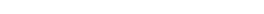.
It measures the amount of "stuff" flowing through a surface per unit time in a "stuff fluid" of density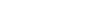moving with local velocity F. Its exterior derivative
Exterior derivative
In differential geometry, the exterior derivative extends the concept of the differential of a function, which is a 1-form, to differential forms of higher degree. Its current form was invented by Élie Cartan....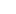is then given by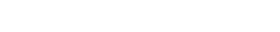Thus, the divergence of the vector field F can be expressed as: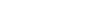Here the superscript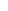is one of the two musical isomorphism
Musical isomorphism
In mathematics, the musical isomorphism is an isomorphism between the tangent bundle TM and the cotangent bundle T*M of a Riemannian manifold given by its metric. There are similar isomorphisms on symplectic manifolds....

s, andis the Hodge dual
Hodge dual
In mathematics, the Hodge star operator or Hodge dual is a significant linear map introduced in general by W. V. D. Hodge. It is defined on the exterior algebra of a finite-dimensional oriented inner product space.-Dimensions and algebra:...

. Note however that working with the current two form itself and the exterior derivative is usually easier than working with the vector field and divergence, because unlike the divergence, the exterior derivative commutes with a change of (curvilinear) coordinate system.

## Generalizations

The divergence of a vector field can be defined in any number of dimensions. If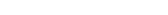in a Euclidean coordinate system where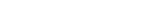and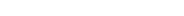, define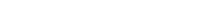The appropriate expression is more complicated in curvilinear coordinates.

For any n, the divergence is a linear operator, and it satisfies the "product rule"for any scalar-valued function.

The divergence can be defined on any manifold of dimension n with a volume form
Volume form
In mathematics, a volume form on a differentiable manifold is a nowhere-vanishing differential form of top degree. Thus on a manifold M of dimension n, a volume form is an n-form, a section of the line bundle Ωn = Λn, that is nowhere equal to zero. A manifold has a volume...

(or density
Density on a manifold
In mathematics, and specifically differential geometry, a density is a spatially varying quantity on a differentiable manifold which can be integrated in an intrinsic manner. Abstractly, a density is a section of a certain trivial line bundle, called the density bundle...

)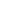e.g. a Riemannian
Riemannian manifold
In Riemannian geometry and the differential geometry of surfaces, a Riemannian manifold or Riemannian space is a real differentiable manifold M in which each tangent space is equipped with an inner product g, a Riemannian metric, which varies smoothly from point to point...

or Lorentzian manifold. Generalising the construction of a two form for a vector field on, on such a manifold a vector field X defines a n-1 form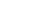obtained by contracting X with. The divergence is then the function defined by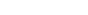Standard formulas for the Lie derivative
Lie derivative
In mathematics, the Lie derivative , named after Sophus Lie by Władysław Ślebodziński, evaluates the change of a vector field or more generally a tensor field, along the flow of another vector field...

allow us to reformulate this as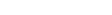This means that the divergence measures the rate of expansion of a volume element as we let it
flow with the vector field.

On a Riemannian or Lorentzian manifold the divergence with respect to the metric volume form
can be computed in terms of the Levi Civita connection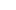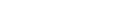where the second expression is the contraction of the vector field valued 1 -form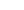with itself and the last expression is the traditional coordinate expression used by physicists.

Divergence can also be generalised to tensors. In Einstein notation
Einstein notation
In mathematics, especially in applications of linear algebra to physics, the Einstein notation or Einstein summation convention is a notational convention useful when dealing with coordinate formulae...

, the divergence of a contravariant vector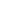is given by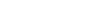where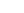is the covariant derivative
Covariant derivative
In mathematics, the covariant derivative is a way of specifying a derivative along tangent vectors of a manifold. Alternatively, the covariant derivative is a way of introducing and working with a connection on a manifold by means of a differential operator, to be contrasted with the approach given...

. Equivalently, some authors define the divergence of any mixed tensor
Mixed tensor
In tensor analysis, a mixed tensor is a tensor which is neither strictly covariant nor strictly contravariant; at least one of the indices of a mixed tensor will be a subscript and at least one of the indices will be a superscript ....

by using the "musical notation #":

If T is a (p,q)-tensor
Tensor
Tensors are geometric objects that describe linear relations between vectors, scalars, and other tensors. Elementary examples include the dot product, the cross product, and linear maps. Vectors and scalars themselves are also tensors. A tensor can be represented as a multi-dimensional array of...

,(p for the contravariant vector and q for the covariant one), then we define the divergence of T to be the (p,q-1)-tensor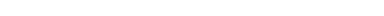,that is we trace the covariant derivative on the first two covariant indices.

• Divergence theorem
Divergence theorem
In vector calculus, the divergence theorem, also known as Gauss' theorem , Ostrogradsky's theorem , or Gauss–Ostrogradsky theorem is a result that relates the flow of a vector field through a surface to the behavior of the vector field inside the surface.More precisely, the divergence theorem...

• Curl